#### You may also like### Kissing Triangles

Determine the total shaded area of the 'kissing triangles'.### Isosceles

Prove that a triangle with sides of length 5, 5 and 6 has the same area as a triangle with sides of length 5, 5 and 8. Find other pairs of non-congruent isosceles triangles which have equal areas.Four rods, two of length a and two of length b, are linked to form a kite. The linkage is moveable so that the angles change. What is the maximum area of the kite?

# Isometric Areas

##### Age 11 to 14Challenge Level
Aditya, Stephanie, Anna, Ahan, Kannammai and Nastassia from West Island School in Hong Kong, Jonathan and Alex and Vaneeza, Miranda, Shafi and Mahnoo from Greenacre Public School in Australia and Pavi, Sofia and Ayana from International School of Lausanne in Switzerland correctly found the areas of the parallelograms.

A: 16 T
B: 24 T
C: 24 T
D: 4 T
E: 16 T
F: 18 T
G: 8 T
H: 12 T

This is the diagram that Matt from Swindon Academy in the UK used to show how triangles of area $1T$ can be drawn inside the parallelograms.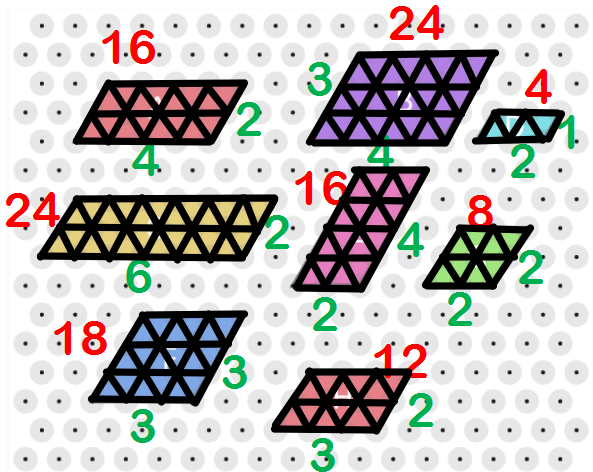Clara from Bangkok Patana School in Thailand found the areas using larger triangles:
To find the area in $T$ units in all of the parallelograms you can simply count the amount of equilateral $T$ triangles in the shape. This however would take a long time so I realised that in a lot of the shapes there can fit a few of the same triangle just scale up by $4T$. I called these triangles '$t$' (lowercase).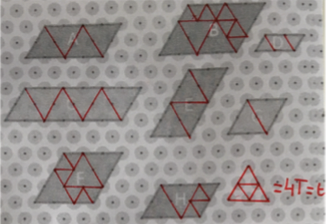Emily from Wycombe High School in the UK, Matt from Swindon Academy in the UK, Noraisha, Jemma, Andre, Piyush, Swiss, Caitlin, Aditya, Anna and Ahan from West Island School in Hong Kong, Vaneeza, Miranda, Shafi and Mahnoo from Greenacre Public School in Australia and Pavi, Sofia and Ayana from International School of Lausanne in Switzerland all noticed that to find the area of a parallelogram in terms of $T$, you need to multiply the lengths of the sides and mutliply by 2.

Kannammai from West Island School in Hong Kong looked at the sides of the parallelograms in terms of 'bases' of the triangles with area $T$. This gives an insight into why the formula works.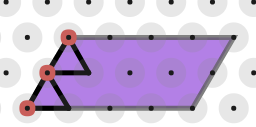If each triangle's base is equal to 1, and if you count there should be 2 bases for side (a) If we do the same but for the top side (b) the length should be 4.

Drawing in the 4 triangles whose bases make the top side (b), as shown below, notice that they don't overlap at all with the 2 triangles along side (a).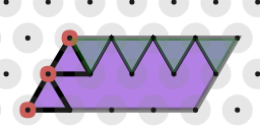This means that inside the parallelogram are 4 sets of 2 triangles the same way around as those along side (a), and 2 rows of 4 triangles the same way round as those along side (b), giving a total of 2$\times$4+4$\times$2 = 16 triangles.

Aditya, Matt, Andre, Piyush, Swiss, Caitlin and Jemma explained the formula by thinking about units. Andre said:
b$\times$h$\times$2 = amount of '$T$s' in the parallelogram because b$\times$h = the amount of  'squares' in the parallelogram and because triangles have half the area of squares we need to multiply (b$\times$h) by 2.

Catilin said:
On isometric paper the units are "$T$", which are triangles. To get the area of a triangle you do b x h $\div$ 2, whereas for squares you simply do b $\times$ h. Therefore, because the units are "$T$" you would have to double the number of triangles as it is only half.

Finding the areas of Trapeziums
This is Matt's diagram for counting the triangles of area $T$ inside a trapezium.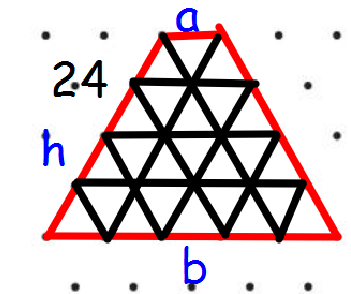Anna, Megha and Roshni from North London Collegiate School split the trapeziums into smaller shapes: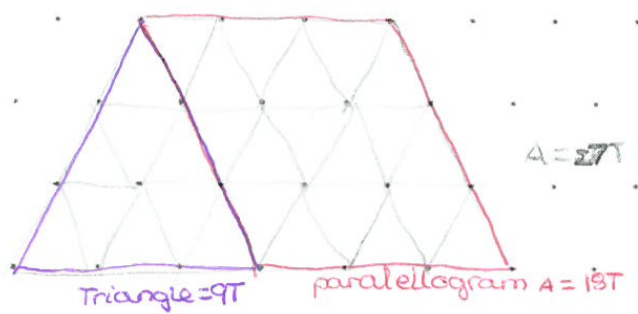With trapeziums, the area is the same as any other parallelogram except you have to add the area of a triangle on to the area. The area of the triangle is also the area of the parallelogram. In this case, $9\times2=18$.

Matt, Caitlin, Swiss, Jemma, Ahan, Kannammai and Pavi, Sofia and Ayana found that the formula for the area of a trapezium in $T$ units is $(a+b)\times h$.

Jemma related this to the formula for the area of a trapezium in normal units:
This is because usually the formula would be ((Base A + Base B) x H) Divided by 2. However because the isometric triangle is half the normal square then you would times two and it would cancel the divided two out.

Pavi, Sofia and Ayana used a diagram to help explain why the formula works: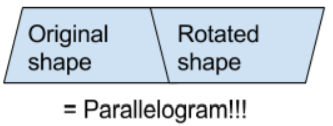We think this works because if you take a trapezium, and you turn it 180$^\text o$ and place it next to the original trapezium, you have a parallelogram! Now here is what it would look like:

So as seen before, the sides of the parallelogram are equal to the height of the trapezium $h$ and the sum of the parallel sides, $a+b$. That means the area of the parallelogram is $2\times h\times(a+b)$ (from the working above), so the area of each trapezium must be $h\times (a+b)$.